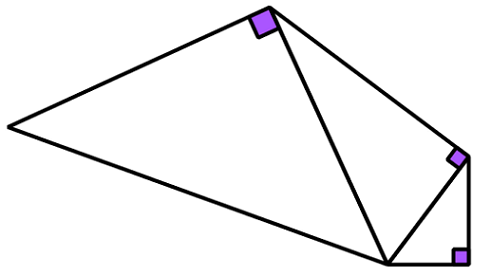# Stacking Right Triangles

Geometry Level 2The above diagram shows three right triangles, each with all integer lengths being stacked on one another.

If the length of the smallest side of the smallest triangle is 3,
what is the length of the largest side of the largest triangle?

Note: Diagram not drawn up to scale.

×